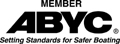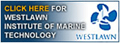Disclaimer:   I am not a spokesperson for the US Coast Guard or ABYC. For an official interpretation of regulations or standards you must contact the US Coast Guard or other organization referenced..   More.....
The entire electrical section of this website may be purchased and downloaded. It is in PDF format and payment is through PAYPAL Go to the store here \$12.50 USD
If you know little or nothing about electrical circuits go to,
Basic Electricity 1  2 3 4 5 6 7 8 9 10 11 12 13 14 15 16
US Navy Basic Electricity Course  or  ABYC Basic Electrical Course
Contact ABYC and the Coast Guard to get the latest standards for Electrical systems.

## ELECTRICAL SYSTEM - Wire Size

### Electrical regulations and standards that apply to boat builders and recreational boats.  Calculating Wire Size .

The following are not verbatim from the regulations. It is my own wording. Get a copy and read the regulation!
The US Coast Guard Electrical Regulations 33 CFR Subpart I sec 183-401 - 183.460 http://newboatbuilders.com/pages/33CFR_electrical.html

How to Calculate Wire Size: These are only examples and each installation will differ.

Before I start, I will define a few terms that you need to know.

Volts:  A unit of measurement of force, or pressure, in an electrical circuit. The common voltage of an AC power line is 120 volts of alternating current (alternating directions). Common voltages used on boats are 12 volts DC and 120 Volts AC.

Amperage(amps): the strength of an electrical current measured in amperes.

Watts: A measure of power. Watts are found by multiplying volts times Amps: For example 120 volts times 1 amp equals 120 watts, which is about the amount of power to light a 100 watt light bulb.

Ohms:  A measure of resistance to the flow of electrical current. One ohm equals one volt divided by one amp. 120 volts divided by 1 amp would be a resistance of 120 ohms.

Load:  The load is the electrical equipment on the circuit. Each item draws a certain amount of amps. The amperage is the load for that circuit. If you add up the amps for each device the total is the total load.

So what does all this mean to you?  If you have a small boat much under 25 feet, it probably has a 12 volt electrical system, which means one, or two batteries.   Most small boats have one battery for lighting the navigation lights, starting the engine, running the depth sounder, the instruments, and bilge pump.   If you have a bass boat you may have a second battery for running the electric trolling motor. Each of these pieces of equipment runs on 12 volts, but they draw different amounts of current or amperes. How do you know how many amperes they need?  Most electrical equipment is rated in Watts. So you can easily find the amperes by dividing watts by volts. Example:  A 300 watt depth sounder needs, 300/12 = 25 amps.

Wire sizing:

You need to know the amperage of a circuit to calculate the wire size for that circuit.  If that circuit has only one load, or piece of equipment on it then selecting the wire size is easy.

First select the temperature rating of the conductor.  What is temperature rating?  Wire comes in different temperature ratings depending on what temperatures it can be exposed to.  This is the rating for the insulation on the wire, not the wire itself. It is rated in degrees C (Celsius).  But it is simpler than that. Most boat manufacturers use 105 degree C wire. So stick with 105.

Next, will the wire be traveling through the engine room, where it can get pretty hot?  If so then there is a factor that needs to be figured in to compensate for the elevated temperature.

Suppose you have one circuit, with one load, a depth sounder, and the wire doesn't go through the engine room. Look at the table and find the column for 105 degrees.  http://newboatbuilders.com/pages/elect2.html Our depth sounder needs 25 amps. Look down the 105 deg. column for 25 amps under the column labeled "outside engine space". If 25 amps is not found go to the next higher number.  Then move over to the left to the first column that says at the top, conductor size.  In this case, 16 gauge.   If the wire had been in the engine room the wire size would have been 12 gauge.   Under each column for temperature rating is a column that says "outside engine space" and "inside engine space". The people who made this table have done the calculation for you that compensates for the hotter engine space.

Now suppose you have more than one load on this circuit.  Suppose you have the depth sounder,  your instrument panel and a marine VHF radio.  You simply add up the amount of amps for each device.  Depth sounder 25 amps.  Instrument panel  3 amps,  radio 5 amps. 25 + 3 + 5 = 33 amps. Then add 10% to the total.  That is 33 + 3.3 = 36.3. Round it up to 37 amps.  Consult the table again. This time the wire size would be 12 gauge.  Why?.  Because 30 amps is a 14 gauge wire, but since you are drawing more than 30 you use the next larger wire size which is 12 gauge.

Now, what about the length of the wire? First you need to know the length of the conductor from the power source to the device. If there is more than one device, then measure along the wire the distance to the one that is farthest away and back. For this you need another table that calculates a 10% voltage drop. http://newboatbuilders.com/pages/elect4.html  Suppose the total length of the wire is 25 feet. Then the table would show that you should select 10 gauge rather than 12 gauge, that is, one size larger.

I have only done a very simple example.  If you to create a more complex system then you need to hire a marine electrician or a consultant to help you design the system. If you are going to have both a DC and AC system you need to consult ABYC http://abycinc.org and hire an electrical engineer to design the system for you.
See
Electrical Planning.  http://newboatbuilders.com/pages/electrical_planning.html

There are on-line calculators that do wire size calculations,  but you should know why it is selecting that wire size before you use them.

Energy Matters DC Cable Sizing Calculator http://www.energymatters.com.au/climate-data/cable-sizing-calculator.php
Energy Development Cooperative LTD Cable Size Calculator Tool - DC Power Cables http://www.solar-wind.co.uk/cable-sizing-DC-cables.html